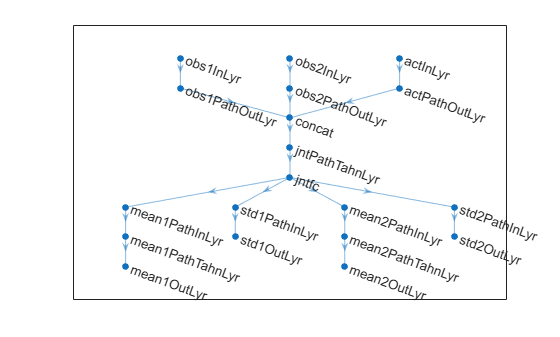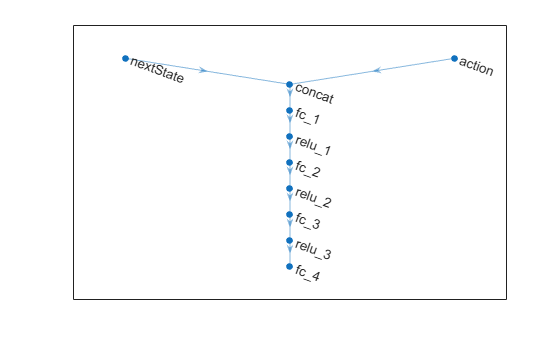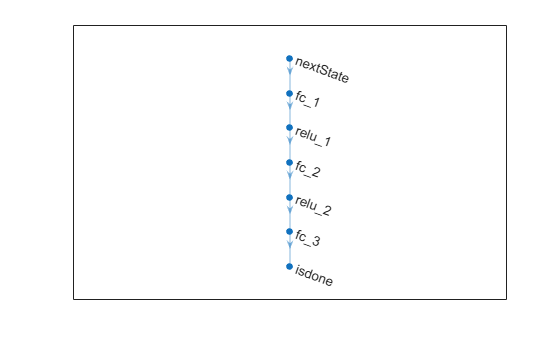# predict

Predict next observation, next reward, or episode termination given observation and action input data

## Syntax

``predNextObs = predict(tsnFcnAppx,obs,act)``
``predReward = predict(rwdFcnAppx,obs,act,nextObs)``
``predIsDone = predict(idnFcnAppx,obs,act)``

## Description

example

````predNextObs = predict(tsnFcnAppx,obs,act)` evaluates the environment transition function approximator object `tsnFcnAppx`, and returns the predicted next observation `nextObs`, given the current observation `obs` and the action `act`.```

example

````predReward = predict(rwdFcnAppx,obs,act,nextObs)` evaluates the environment reward function approximator object `rwdFcnAppx`, and returns the predicted reward `predReward`, given the current observation `obs`, the action `act`, and the next observation `nextObs`.```

example

````predIsDone = predict(idnFcnAppx,obs,act)` evaluates the environment is-done function approximator object `idnFcnAppx`, and returns the predicted is-done status `predIsDone`, given the current observation `obs`, the action `act`, and the next observation `nextObs`.```

## Examples

collapse all

Create observation and action specification objects (or alternatively use `getObservationInfo` and `getActionInfo` to extract the specification objects from an environment). For this example, there are two observation channels carrying vectors in a four and two-dimensional space, respectively. The action is a continuous three-dimensional vector.

```obsInfo = [rlNumericSpec([4 1],'UpperLimit',10*ones(4,1)); rlNumericSpec([1 2],'UpperLimit',20*ones(1,2)) ]; actInfo = rlNumericSpec([3 1]);```

Create a deep neural network to be used as approximation model for the transition function approximator. For a continuous Gaussian transition function approximator, the network must have two output layers for each observation (one for the mean values the other for the standard deviation values).

```% input path layers from first observation channel inPath1 = [ featureInputLayer(prod(obsInfo(1).Dimension), ... 'Normalization','none','Name','netObsIn1') fullyConnectedLayer(5,'Name','infc1') ]; % input path layers from second observation channel inPath2 = [ featureInputLayer(prod(obsInfo(2).Dimension), ... 'Normalization','none','Name','netObsIn2') fullyConnectedLayer(5,'Name','infc2') ]; % input path layers from action channel inPath3 = [ featureInputLayer(prod(actInfo(1).Dimension), ... 'Normalization','none','Name','netActIn') fullyConnectedLayer(5,'Name','infc3') ]; % joint path layers, concatenate 3 inputs along first dimension jointPath = [ concatenationLayer(1,3,'Name','concat') tanhLayer('Name','tanhJnt'); fullyConnectedLayer(10,'Name','jntfc') ]; % path layers for mean values of first predicted obs % using scalingLayer to scale range from (-1,1) to (-10,10) % note that scale vector must be a column vector meanPath1 = [ tanhLayer('Name','tanhMean1'); fullyConnectedLayer(prod(obsInfo(1).Dimension)); scalingLayer('Name','scale1', ... 'Scale',obsInfo(1).UpperLimit) ]; % path layers for standard deviations first predicted obs % using softplus layer to make them non negative sdevPath1 = [ tanhLayer('Name','tanhStdv1'); fullyConnectedLayer(prod(obsInfo(1).Dimension)); softplusLayer('Name','splus1') ]; % path layers for mean values of second predicted obs % using scalingLayer to scale range from (-1,1) to (-20,20) % note that scale vector must be a column vector meanPath2 = [ tanhLayer('Name','tanhMean2'); fullyConnectedLayer(prod(obsInfo(2).Dimension)); scalingLayer('Name','scale2', ... 'Scale',obsInfo(2).UpperLimit(:)) ]; % path layers for standard deviations second predicted obs % using softplus layer to make them non negative sdevPath2 = [ tanhLayer('Name','tanhStdv2'); fullyConnectedLayer(prod(obsInfo(2).Dimension)); softplusLayer('Name','splus2') ]; % add layers to network object net = layerGraph; net = addLayers(net,inPath1); net = addLayers(net,inPath2); net = addLayers(net,inPath3); net = addLayers(net,jointPath); net = addLayers(net,meanPath1); net = addLayers(net,sdevPath1); net = addLayers(net,meanPath2); net = addLayers(net,sdevPath2); % connect layers net = connectLayers(net,'infc1','concat/in1'); net = connectLayers(net,'infc2','concat/in2'); net = connectLayers(net,'infc3','concat/in3'); net = connectLayers(net,'jntfc','tanhMean1/in'); net = connectLayers(net,'jntfc','tanhStdv1/in'); net = connectLayers(net,'jntfc','tanhMean2/in'); net = connectLayers(net,'jntfc','tanhStdv2/in'); % plot network plot(net)``````% convert to dlnetwork net=dlnetwork(net);```

Create a continuous Gaussian transition function approximator object, specifying the names of all the input and output layers.

```tsnFcnAppx = rlContinuousGaussianTransitionFunction(... net,obsInfo,actInfo,... ObservationInputNames={'netObsIn1','netObsIn2'}, ... ActionInputNames='netActIn', ... NextObservationMeanOutputNames={'scale1','scale2'}, ... NextObservationStandardDeviationOutputNames={'splus1','splus2'} );```

Predict the next observation for a random observation and action.

```predObs = predict(tsnFcnAppx, ... {rand(obsInfo(1).Dimension),rand(obsInfo(2).Dimension)}, ... {rand(actInfo(1).Dimension)})```
```predObs=1×2 cell array {4x1 single} {[-24.9934 0.9501]} ```

Each element of the resulting cell array represents the prediction for the corresponding observation channel.

To display the mean values and standard deviations of the Gaussian probability distribution for the predicted observations, use `evaluate`.

```predDst = evaluate(tsnFcnAppx, ... {rand(obsInfo(1).Dimension),rand(obsInfo(2).Dimension), ... rand(actInfo(1).Dimension)})```
```predDst=1×4 cell array {4x1 single} {2x1 single} {4x1 single} {2x1 single} ```

The result is a cell array in which the first and second element represent the mean values for the predicted observations in the first and second channel, respectively. The third and fourth element represent the standard deviations for the predicted observations in the first and second channel, respectively.

Create an environment interface and extract observation and action specifications. Alternatively, you can create specifications using `rlNumericSpec` and `rlFiniteSetSpec`.

```env = rlPredefinedEnv("CartPole-Continuous"); obsInfo = getObservationInfo(env); actInfo = getActionInfo(env);```

Create a deep neural network. For this example, the network has two input channels, one for the current action and one for the next observations. The single output channel contains a scalar, which represents the value of the predicted reward.

```actionPath = featureInputLayer(actInfo.Dimension(1), ... Normalization="none",Name="action"); nextStatePath = featureInputLayer(obsInfo.Dimension(1), ... Normalization="none",Name="nextState"); commonPath = [concatenationLayer(1,2,Name="concat") fullyConnectedLayer(64,Name="FC1") reluLayer(Name="CriticRelu1") fullyConnectedLayer(64,Name="FC2") reluLayer(Name="CriticCommonRelu2") fullyConnectedLayer(64,Name="FC3") reluLayer(Name="CriticCommonRelu3") fullyConnectedLayer(1,Name="reward")]; rewardNetwork = layerGraph(nextStatePath); rewardNetwork = addLayers(rewardNetwork,actionPath); rewardNetwork = addLayers(rewardNetwork,commonPath); rewardNetwork = connectLayers(rewardNetwork,"nextState","concat/in1"); rewardNetwork = connectLayers(rewardNetwork,"action","concat/in2"); plot(rewardNetwork)```Create `dlnetwork` object.

`rewardNetwork = dlnetwork(rewardNetwork);`

Create deterministic transition function object.

```rewardFcnAppx = rlContinuousDeterministicRewardFunction(... rewardNetwork,obsInfo,actInfo,... ActionInputNames="action", ... NextObservationInputNames="nextState");```

Using this reward function object, you can predict the next reward value based on the current action and next observation. For example, predict the reward for a random action and next observation. Since, for this example, only the action and the next observation influence the reward, use an empty cell array for the current observation.

```act = rand(actInfo.Dimension); nxtobs = rand(obsInfo.Dimension); reward = predict(rewardFcnAppx,{}, {act}, {nxtobs})```
```reward = single 0.1034 ```

To predict the reward, you can also use `evaluate.`

`reward_ev = evaluate(rewardFcnAppx, {act,nxtobs} )`
```reward_ev = 1x1 cell array {[0.1034]} ```

Create an environment interface and extract observation and action specifications. Alternatively, you can create specifications using `rlNumericSpec` and `rlFiniteSetSpec`.

```env = rlPredefinedEnv("CartPole-Continuous"); obsInfo = getObservationInfo(env); actInfo = getActionInfo(env);```

Create a deep neural network. The network has one input channel for the next observations. The single output channel is for the predicted termination signal.

```commonPath = [featureInputLayer(obsInfo.Dimension(1), ... Normalization="none",Name="nextState"); fullyConnectedLayer(64,Name="FC1") reluLayer(Name="CriticRelu1") fullyConnectedLayer(64,Name="FC3") reluLayer(Name="CriticCommonRelu2") fullyConnectedLayer(2,Name="isdone0") softmaxLayer(Name="isdone")]; isDoneNetwork = layerGraph(commonPath); plot(isDoneNetwork)```Covert the network to a `dlnetwork` object.

`isDoneNetwork = dlnetwork(isDoneNetwork);`

Create an is-done function approximator object.

```isDoneFcnAppx = rlIsDoneFunction(... isDoneNetwork,obsInfo,actInfo,... NextObservationInputNames="nextState");```

Using this is-done function approximator object, you can predict the termination signal based on the next observation. For example, predict the termination signal for a random next observation. Since for this example the termination signal only depends on the next observation, use empty cell arrays for the current action and observation inputs.

```nxtobs = rand(obsInfo.Dimension); predIsDone = predict(isDoneFcnAppx,{},{},{nxtobs})```
```predIsDone = 0 ```

You can obtain the termination probability using `evaluate`.

`predIsDoneProb = evaluate(isDoneFcnAppx,{nxtobs})`
```predIsDoneProb = 1x1 cell array {2x1 single} ```
`predIsDoneProb{1}`
```ans = 2x1 single column vector 0.5405 0.4595 ```

The first number is the probability of obtaining a 0 (no termination predicted), the second one is the probability of obtaining a 1 (termination predicted).

## Input Arguments

collapse all

Environment transition function approximator object, specified as one of the following:

Environment reward function approximator object, specified as one of the following:

Environment is-done function approximator object, specified as an `rlIsDoneFunction` object.

Observations, specified as a cell array with as many elements as there are observation input channels. Each element of `obs` contains an array of observations for a single observation input channel.

The dimensions of each element in `obs` are MO-by-LB, where:

• MO corresponds to the dimensions of the associated observation input channel.

• LB is the batch size. To specify a single observation, set LB = 1. To specify a batch of observations, specify LB > 1. If `valueRep` or `qValueRep` has multiple observation input channels, then LB must be the same for all elements of `obs`.

LB must be the same for both `act` and `obs`.

For more information on input and output formats for recurrent neural networks, see the Algorithms section of `lstmLayer`.

Action, specified as a single-element cell array that contains an array of action values.

The dimensions of this array are MA-by-LB, where:

• MA corresponds to the dimensions of the associated action specification.

• LB is the batch size. To specify a single observation, set LB = 1. To specify a batch of observations, specify LB > 1.

LB must be the same for both `act` and `obs`.

For more information on input and output formats for recurrent neural networks, see the Algorithms section of `lstmLayer`.

Next observations, that is the observation following the action `act` from the observation `obs`. It is a cell array of the same dimension as `obs`.

## Output Arguments

collapse all

Predicted next observation, that is the observation predicted by the transition function approximator `tsnFcnAppx` given the current observation `obs` and the action `act`. It is an cell array of the same dimension as `obs`.

Predicted reward, that is the reward predicted by the reward function approximator `rwdFcnAppx` given the current observation `obs`, the action `act`, and the following observation `nextObs`.

Predicted is-done episode status, that is the episode termination status predicted by the is-done function approximator `rwdFcnAppx` given the current observation `obs`, the action `act`, and the following observation `nextObs`.

Note

If `fcnAppx` is an `rlContinuousDeterministicRewardFunction`, then `predict` behaves identically to `evaluate` except that `evaluate` returns results inside a single-cell array. If `fcnAppx` is an `rlContinuousDeterministicTransitionFunction` object, then `predict` behaves identically to `evaluate`. If `fcnAppx` is an `rlContinuousGaussianTransitionFunction` then `evaluate` returns the mean value and standard deviation the observation probability distribution, while `predict` returns an observation sampled from this distribution. Similarly, for an `rlContinuousGaussianRewardFunction` object, `evaluate` returns the mean value and standard deviation the reward probability distribution, while `predict` returns a predicted reward sampled from this distribution. Finally, if `fcnAppx` is an `rlIsDoneFunction` object, then `evaluate` returns the probabilities of the termination status being false or true, respectively, while `predict` returns a predicted termination status sampled with these probabilities.

## Version History

Introduced in R2022a